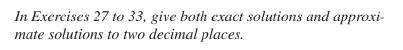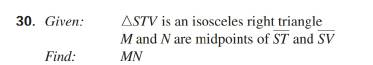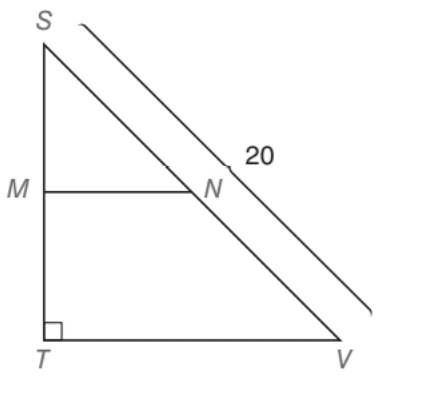Chapter 5.5, Problem 30EElementary Geometry For College St...

7th Edition
Alexander + 2 others
ISBN: 9781337614085

Solutions

Chapter
SectionElementary Geometry For College St...

7th Edition
Alexander + 2 others
ISBN: 9781337614085
Textbook ProblemTo determine

To find:

MN of the isosceles right triangle STV with M and N are the midpoints of ST¯ and SV¯.

Explanation

Approach:

For a right triangle for which the measure of the interior angles 45°, 45°, and 90°, if ‘a’ is the length of measure of one of the leg; opposite to the angle 45°, then the length of the other two sides is given by

Length of the other leg =a

Length of the hypotenuse =a2

In general

Length of the legs are equal.

Length of the hypotenuse =2× (Length of one of the legs)

Calculation:

Given,

STV is an isosceles triangle with right angle at T.

M and N are the midpoints of ST¯ and SV¯.

SV=20 units.

Since, STV is an isosceles right , two of the sides must be congruent other than the hypotenuse.

From the given diagram SV¯.is the hypotenuse.

Thus,

TS¯TV¯

Since, two of the legs of the right STV are congruent, the angle opposite to those congruent sides must be congruent.

Hence, STV is of the 45°-45°-90° type of right triangle.

By the application of the 45-45-90 theorm,

45°-45°-90° theorem.

In a right triangle whose angle measures 45°, 45°, and 90°, the legs are congruent and the hypotenuse has a length equal to the product of 2 and the length of either leg

Still sussing out bartleby?

Check out a sample textbook solution.

See a sample solution

The Solution to Your Study Problems

Bartleby provides explanations to thousands of textbook problems written by our experts, many with advanced degrees!

Get Started

Convert the expressions in Exercises 8596 radical form. x4/3

Finite Mathematics and Applied Calculus (MindTap Course List)

In problems 11-16, write in radical form.Do not simplify. 12.

Mathematical Applications for the Management, Life, and Social Sciences

Evaluate the integral. 22. costdt

Single Variable Calculus: Early Transcendentals

What does the expected value of a game measure?

Finite Mathematics for the Managerial, Life, and Social Sciences

The graph of θ = 2 in polar coordinates is a: circle line spiral 3-leaved rose

Study Guide for Stewart's Single Variable Calculus: Early Transcendentals, 8th

True or False:

Study Guide for Stewart's Multivariable Calculus, 8th